American Journal of Modern Physics
Volume 5, Issue 1, January 2016, Pages: 15-19

Crystallization of Hard-Sphere Assembly of Fermions

Samuel Limo Chelimo1, *, Khanna Mohan Kapil1, Joel Kipkorir Tonui1, Godfrey Sylvanous Murunga1,
Joshua Kiprotich Kibet2

1Department of Physics, University of Eldoret, Eldoret, Kenya

2Department of Chemistry, Egerton University, Egerton, Kenya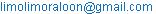(S. L. Chelimo)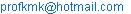(K. M. Khanna)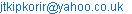(J. K. Tonui)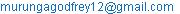(G. S. Murunga)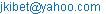(J. K. Kibet)

Samuel Limo Chelimo, Khanna Mohan Kapil, Joel Kipkorir Tonui, Godfrey Sylvanous Murunga, Joshua Kiprotich Kibet. Crystallization of Hard-Sphere Assembly of Fermions. American Journal of Modern Physics. Vol. 5, No. 1, 2016, pp. 15-19. doi: 10.11648/j.ajmp.20160501.12

Abstract: Crystallization of a hard-sphere system of fermions with densities ranging from low to high values has been studied. Saturation densities at which the total energy E, is maximum has been calculated. The values of saturation particle number densities ps for low and high densities are; 7.11x1021 particles/cm3 and 1.502x1023 particles/cm3 respectively at which the fermions close pack or crystallize. Variation of pswith hard-sphere diameter C is not linear and it is more or less the same for both low and high density since crystallization occurs in both the cases. The total energy, E, has been found to vary non-linearly with p at high densities and closely linear for low density. The value of E for low density is 1.435x10-22 J, and for high density it is 3.113x10-21 J. These findings are consistent with experimental and computer-simulated results obtained by others.

Keywords: Fermions, Crystallization, Saturation Density

Contents

1. Introduction

In general, for physical systems, whether molecular or nuclear, the interaction potential is composed of repulsive part and attractive part. The most illustrative such potential for molecular systems is the Lennard-Jones potential. Calculations have been done using potential composed of a finite repulsive part followed by an attractive part, and also using infinite repulsive part followed by a finite attractive part. Physical systems by themselves do not freeze or crystallize. Reduction of temperature followed by the application of large external pressure can lead to freezing and or crystallization. By implication, this means that when the two particles come closer, they tend to repel each other within some inter-particle distance, and unless this repulsion is overcome, they cannot constitute a motionless crystalline structure. It is this way of looking at the problem that motivated us to consider that the interaction between two particles coming very close to each other could be a hard sphere one. The system of particles that has been chosen is a Fermi system.

Fermions are particles with half-integral spin. To decide whether an atom is a fermion, we count the total number of protons, neutrons and electrons constituting the atom, and if the number is odd, the atom will be a fermion since each constituent of the atom has spin 1/2, and each is a fermion by itself. Two indistinguishable fermions can never occupy the same quantum state due to Pauli’s exclusion principle. But when two fermions collide, the S-wave cross-section diverges at zero energy, and the p-wave cross-section goes to zero. The scattering amplitude at zero energy equals minus the scattering length.

The S-wave scattering is repulsive at low energies, while the p-wave scattering is attractive. For S-wave contact interaction when the interaction will be infinitely repulsive, hard-sphere or hard-core type interaction, the S-wave scattering length as will be equal to the hard-sphere diameter ‘C’. In fact, in an assembly of fermions in which pairs of particles interact via a hard-sphere repulsive core as=C which is also called the range of interaction. In what follows, we study the properties of a hard-sphere assembly of fermions. It was found  that in the classical hard-sphere system a freezing transition from a fluid to crystalline phase can take place as the density of the system is increased. Thus it is quite possible that the same phenomena can occur in quantum systems, whether bosons are fermions; and on crystallization or close packing, distinction between bosons and fermions disappears.

The properties of hard-sphere assembly of crystalline bosons have been studied earlier . To obtain the properties of an assembly of hard-sphere fermions, it is well known that fermions have half integral spin and obey Fermi-Dirac statistics. For a non-interacting gas of such particles, in thermal equilibrium at a temperature T<<TF = EF/k, where EF is the Fermi energy, TF is the corresponding Fermi temperature and these values are determined by the mass and density of the gas; then all states lying below EF in energy are occupied by a single particle, and those above EF are empty. The re-arrangement of the particles can take place only in an energy shell of width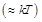around EF, and the thermal, transport and response properties are thus determined by the properties of states in this shell. Landau  had shown that under appropriate conditions, this picture remains qualitatively valid even in the presence of strong inter-particle interactions. The systems are then known as a degenerate Fermi gas or liquid. The experiments showed that the Fermi systems behave as degenerate up to T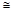100 mK or below. The strong interaction that has been chosen in this study is that of hard-sphere interaction.

In general, in dilute gases of fermions, the pair interactions have a range much smaller than inter-particle spacing . But, when the two-particle scattering length is large (weak interactions), these short range interactions can modify the gas properties sufficiently. Such examples are low density neutron matter that may occur in the inner crust of neutron stars .

Here in this manuscript, the properties of a crystalline Fermi system will be studied. In such a system, the fermions will be very close to each other, and the interaction between a pair of fermions will be assumed to be a hard sphere one.

The hard-sphere system is a useful first approximation to a many-body system via a pair potential containing a short-ranged repulsive part. This description is better at very low densities where the particles experience weakly attractive potential tail surrounding the repulsion or at very high densities where the repulsion is predominant. However, at intermediate densities, the attractive potential may play a significant role.

Therefore, a hard-sphere system of fermions with densities ranging from very low to very high values will be considered to obtain an expression for the energy per particle E/N. Fermions need to satisfy Pauli’s exclusion principle which leads to repulsion between two fermions when they try to approach each other to occupy the same energy state. A gas or liquid composed of 3He and or neutrons is a well known assembly of fermions. For a N-identical fermion system, the total energy E in terms of various parameters has been calculated [5,6]. It was found that in hard-sphere system, freezing transition from a fluid to a crystalline phase occurs as the density is increased. It is, therefore possible that the same phenomena may occur in quantum systems, whether of bosons or fermions . The interaction potential U(r) between a pair of fermions is assumed to be the hard-sphere potential of diameter C, defined as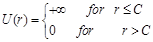(1)

A generalized London equation was  proposed for a N-fermion hard-sphere system with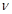intrinsic degrees of freedom for each fermion. For systems like 3He and neutron matter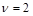but for nuclear matter composed of neutrons and protons,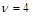. In factis essentially the maximum occupation number in a given single-particle quantum state, and for bosons it is infinite.

Thus the crystallization of hard-sphere system of fermions with densities from low to high values has been studied. Saturation densities leading to crystallization of hard-sphere fermions, and the energy per particle E/N, have been calculated.

2. Theoretical Calculations

A gas or a liquid composed of fermions in which pairs of particles interact via hard-sphere interaction has been studied to obtain the total energy E of the system and to obtain the saturation density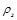, leading to crystallization of the system. The well known fermion systems are 3He, neutron matter and symmetric nuclear matter. The degrees of freedomfor 3He is v=2,for neutron matter v=2, but for symmetric nuclear matter composed of equal number of neutrons and protons v=4. It will be assumed that each particle of diameter, C, is confined to move in a space with the characteristic dimension R, such that the mean particle spacing is R-C. Using Heisenberg’s uncertainty principle, it is easy to show that the energy per particle E/N can be written as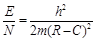(2)

Where m = mass of each particle and if the ultimate particle number density in the space with characteristic dimension R=2.84x10-8cm , then the total number of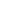particles N, in the system whose volume is V, can be written as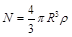(3)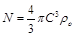(4)

Where= the particle number density at crystallization.

Substituting for R and C from equations (3) and (4) in equation (2), we get E/N in a general form,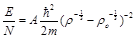(5)

Here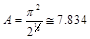is a constant called the residue of the pole at close packing. Theoretically A is predicted  to lie within 1.63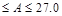for random close packing (rcp) polyhedron cell and for regular close Packing (face centered cubic) or hexagonal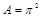. However, experimentally the value of A obtained from the high pressure data of 3He, 4He, H and 2H is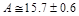for the crystalline branch of the equation of state.

The general expression for uniform fermion hard-sphere systems with a degeneracy factor of v for the ground state energy per particle with a hard-core potential of range C  is close pack at the same densities. The value of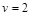for a fermion gas and the reduced mass of 3He is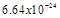gm.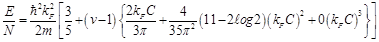(6)

by considering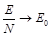, and introducing the thermal activation factor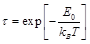into Eqn (6), we can write,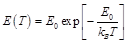(7)

where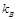is the Boltzmann constant.

This gives the transition temperature for a hard-sphere assembly of fermions, i.e,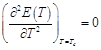(8)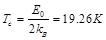(9)

The transition temperature at which phase transition takes place in a system of hard-sphere gas of fermions (3He) is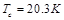. Thus the value of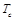obtained in this manuscript for crystallization of fermions and given in Eqn (9) is close to thevalue obtained earlier . However, this value is lower, andcan vary if the density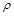varies; and if the hard-sphere diameter C varies.

An improved hard-sphere ground state equation of state for N-fermions hard-spheres  is given by,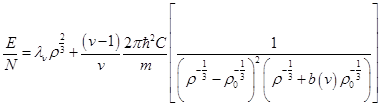(10)

where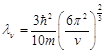,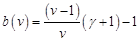and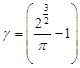Eqn (10) is the generalized modified London equation. In the limit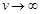,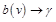and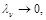then Eqn (10) show

At both low and high densities, Eqn (10) reduces to the limiting expressions.

Low density condition:

At low density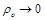, Eqn (9) becomes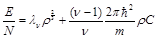(11)

Where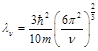High density condition:

At high density,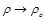and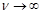in Eqn (9) such that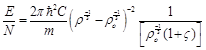(12)

Now using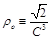and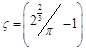, Eqn (12) gives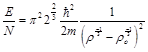(13)

Where π2 22/3 =A=15.7and m is the reduced mass for 3He.

The saturation density for an assembly of fermions will be defined as that density ρs, at which the hard-sphere assembly of fermions form close-pack (cp) crystalline structure. At this density, the total energy, E, of the assembly will be such that,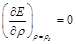(14)

Now using low density value of E/N from Eqn (11) in Eqn (14), we can getas a function of C as,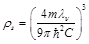(15)

In the high density system, the saturation density is obtained by using Eqn (13) in Eqn (14), and this calculation yields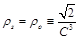(16)

3. Results and Discussions

Starting from the expression for the ground state energy per particle,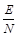of a hard-sphere assembly of fermions, (i) we have studied the variation ofwith density, both for low and high density assemblies, (ii) have studied the variation of saturation density with hard-sphere diameter C both for low density and high density, (iii) have calculated the transition temperatureat which crystallization of fermions can take place.

For a low density assembly (Fig. 1), the value ofvaries linearly with density. This may be due to the fact that at low density the interaction plays less roles in crystallization of fermions and the energy simply increases with particle number.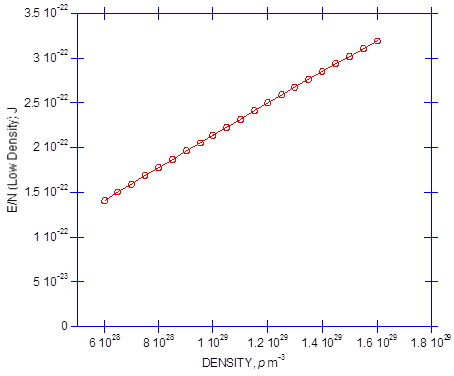Figure 1. Energy per Particle for low Density.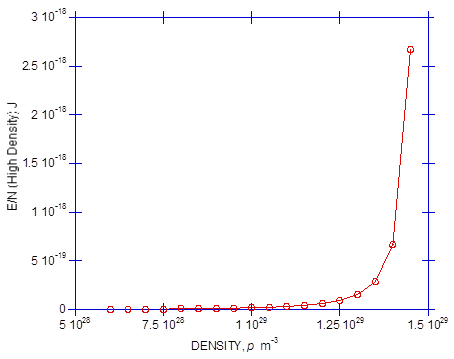Figure 2. Energy per Particle for high Density.

For high density assembly of fermions (Fig. 2), there is no much variation inup to a particular density, and then at some value of density, the value ofsuddenly rises. This could be due to the fact that when the density becomes large, close to ρs, interactions become predominant due to the proximity of the particles at high density, and hence the energysuddenly becomes large.

The variation of saturation density with hard-sphere diameter is shown in Fig. 3. In the case of low density, the value ofvaries relative linearly with C, which means at low density the variation ofwith C is insignificant since the inter-particle distance may be larger than C. But for high density assembly, the value ofincreases sharply as C decreases, and this is an essential condition for crystallization.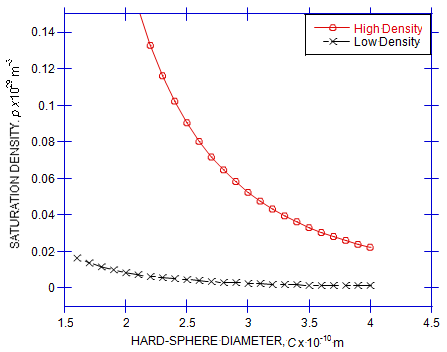Figure 3. Variation of Saturation Density with corresponding Hard-sphere diameter for 3He particles.

Table 1. Energy per particle and Saturation density for low and high densities(Free-particle spacing, R =2.8401 Å).

 Low Density High Density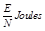1.435x10-22 3.113x10-21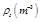7.117x1027 1.502x1029

The transition temperaturewill vary withand C. For our values ofand C,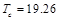K, but this could vary from assembly to assembly. Our results show that for both low and high density; ρs varies as C-3 which means that the assembly will crystallize for some value of C irrespective of the value of ρs. Such results were obtained for bosons by Khanna et al. .

In conclusion, our saturation density,values for low and high density compare well with laboratory, computer-simulation and the variational results [11, 12] and those of Zhizhong et al. .

References

1. J. G. Kirkwood, E. K. Maun and B. J. J. Adler, "Radial distribution functions and the equation of state of a fluid composed of rigid spherical molecules," J. Chem. Phys., vol.18, pp. 1040-1047, 1950.
2. K. M. Khanna, Y. K. Ayodo and T. W. A. Sakwa, "Hard Sphere Assembly of Crystalline Bosons," Afr. Phys. Rev., 2011, 4:0001.
3. L. D. Landau, "Theory of a Fermi Liquid," Zh. Eksp. Teor. Fiz, vol. 30, pp. 1058, 1956. [Sovi. Phys. JETP vol. 3, pp. 920, 1957].
4. G. A. Baker, "Neutral Matter Model," Phys. Rev., vol. C(60), 1999, 054311.
5. C. J. Pethick and D. G. Ravenhall, "Matter at Large Neutron Excess and the Physics of Neutron-Star Crusts," Ann. Rev. Nucl. Part. Sci., vol. 45, pp. 429-484, 1995.
6. G. A. Baker Jr., "Singularity Structure of the Perturbation Series for the Ground-State Energy of a Many-Fermion System," Rev. Mod. Phys., vol. 43, pp. 479, 1971.
7. G. A. Baker Jr., L. P. Benofy, M. de Llano, M. Fortes, S. M. Peltier and A. Pastino, "Hard-core square-well fermions," Phys. Rev., vol. A26, pp. 3575, 1982.
8. M. A. Solìs, M. de Llano and J. W. Clark, "Kirkwood Phase Transition for Boson and Fermion Hard-Sphere Systems," arXiv: cond-mat/0306338v1, 2003.
9. A. L. Fetter and J. D. Walecka, "Quantum Theory of Many-Particle System," Mcgraw-Hill: New York, 1971, pp. 149.
10. S. L. Chelimo, K. M. Khanna, K. K. Sirma, J. K. Tonui, P. K. Korir, J. K. Kibet, A. J. Achieng and A. Sarai, "Quantum Hard-Sphere Assembly of Fermions," Int. J. of Phys. and Math. Sci., vol. 5(1), pp. 47-53, 2015.
11. M. Solis, D. Llano, J. Clark and G. A. Baker, "Improved hard -sphere Ground state Equation of state," Cond-Stat-Mech., 63130, 2013.
12. M. Viviani, E. Bundia, S. Fantoni and S. Rosati, "Spin-dependent correlations in the ground state of liquid Helium-3," Phys. Rev., vol. B38, pp. 4523, 1988.
13. H. M. Zhizhong, D. Llano, E. Bundia and R. Guardiola, "Aziz fermions and liquid 3He," Phys. Rev., vol. B43 (16), 1991.

 Contents 1. 2. 3.
Article ToolsAbstractPDF(333K)